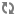Creating Custom Builder Components# Creating UDAFs

User Defined Aggregate Functions (UDAF) allow you to add custom aggregate functions to SAM. Once you create and register UADFs they are available for use in the Aggregate processor. Use these steps to create a new UADF.

1. Create a UADF by implement the following interface:
```public interface UDAF<A, V, R> {
A init();
R result(A aggregate);
}
```

Where:

• A – Is the type of the aggregate that is used to aggregate the values. init returns the initial value for the aggregate.

• V – is the type of the values we are processing. The add method is invoked with the current aggregate and the value for each of the events in the window. add is expected to aggregate the current value and return the updated aggregate.

• R – is the result type and the result function takes the final aggregated value and returns the result.

2. For aggregate functions that requires two parameters, the UDAF2 interface also requires implementation. The only difference is that the add function is passed the current value of the aggregate and two values instead of one.
```public interface UDAF2<A, V1, V2, R> {
A init();
A add(A aggregate, V1 val1, V2 val2);
R result(A aggregate);
}
```

In this example, you want to compute the average values of a particular field for events within a window. To do that, define an average aggregate function by implementing the UDAF interface as shown below:

```// Here the aggregate is a pair that holds the running sum and the count of elements seen so far
// The values are integers and the result is a double.
public class MyAvg implements UDAF<Pair<Integer, Integer>, Integer, Double> {

// Here we initialize the aggregate and return its initial value (sum = 0 and count = 0).
@Override
public Pair<Integer, Integer> init() { return Pair.of(0, 0); }

// Here we update the sum and count values in the aggregate and return the updated aggregate
@Override
public Pair<Integer, Integer> add(Pair<Integer, Integer> agg, Integer val) {
return Pair.of(agg.getKey() + val, agg.getValue() + 1);
}

// Here we return the value of the sum divided by the count which is the average of the aggregated values.
@Override
public Double result(Pair<Integer, Integer> agg) {
return (double) agg.getKey() / agg.getValue();
}
}
```